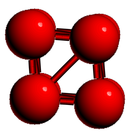Crystal Lattice Structures: Creation Date: 26 Jan 1996 Last Modified: 21 Oct 2004

# The βPo (Ai) StructureYou can now

• This lattice has only rhombohedral symmetry. When the parameter "x" below is set to 1/3 the atoms are on the sites of a face centered cubic lattice, when x = 0 the atoms are on the sites of a simple cubic lattice, and when x = -1/6 the atoms are on the sites of a body centered cubic lattice. By convention, lattices with 0 < x < 1/3 belong to Strukturbericht A10, while lattices with -1/6 < x < 0 belong to Ai.
• The primitive vectors here are oriented to agree with the simple cubic lattice when x = 0.

• Prototype: βPo
• Pearson Symbol: hR1
• Strukturbericht Designation: Ai
• Space Group: R3m (Cartesian and lattice coordinate listings available)
• Number: 166
• Reference: Pearson (Alloys), p. 559.
• Other elements with this Structure: None, but see alpha Hg (A10)
• Primitive Vectors:  A1 = a (1 + x) X + a x Y + a x Z A2 = a x X + a (1 + x) Y + a x Z A3 = a x X + a x Y + a (1 + x) Z
• Basis Vectors:  B1 = 0 (Po) (1a)

### See these vectors in LaTeX output format.

Go back to the simple cubic and related structures page.

Go back to Crystal Lattice Structure page.

 Structures indexed by: This is a mirror of an old page created at theNaval Research LaboratoryCenter for Computational Materials ScienceThe maintained successor is hosted at http://www.aflowlib.org/CrystalDatabase/ and published as M. Mehl et al., Comput. Mater. Sci. 136 (Supp.), S1-S828 (2017).